# Half of my 6* roster is science. What are these odds?

2»

• 4 out of my 5 6 stars are science and all of them are trash besides mr fantastic who is decent
• DNA3000 said:What are the odds of this even happening.

Five of eleven champs all the same class? 12%. The odds of getting at least five of the same class is slightly higher, about 14%.

I could do the calculation(s), but I'm lazy today so it is Wolfram Alpha to the rescue: https://www.wolframalpha.com/input/?i=what+are+the+odds+of+seeing+five+of+a+kind+in+eleven+dice

That's about one in seven, so just for giggles I decided to use an online dice roller and see how long it took to get five of a kind. Here we go: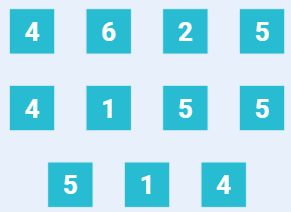.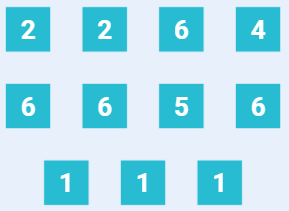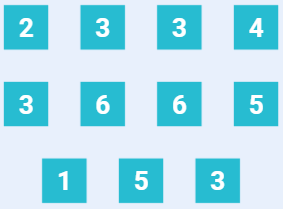.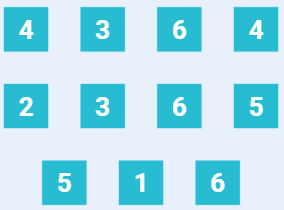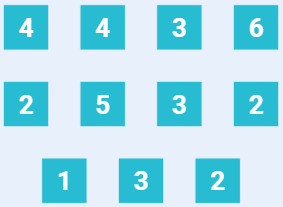.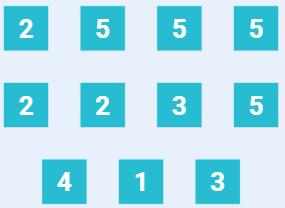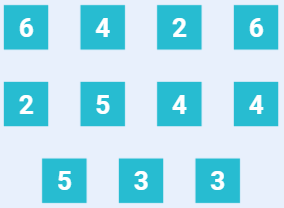.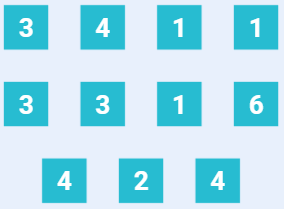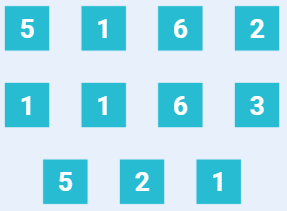.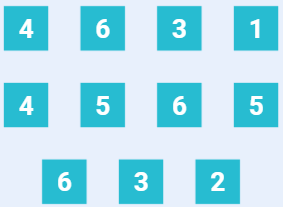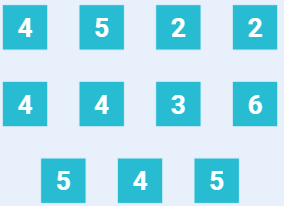.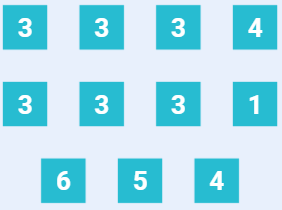It took twelve rolls, but I did get six of a kind. I think it was worth posting these just to see how many people look at these rolls and think they aren't random. Because its been proven repeatedly that humans cannot recognize randomness. They see order, and they see uniformity, but randomness is neither. People see structure in randomness and assume that structure can't be randomly generated. But by definition, random sequences contain all possible structures, just in unpredictable patterns.
How did you get 12%. I used a binomial distribution with 11trials and got around 20%
• Assuming every class has same number of champs (it's most likely not, but if you want, you can post exact number of science champs), let's say Ps = 1/6 - chance to get science champ.

With total of 11 champs, to get 5 of 11 champs in science class and 6 in any other class, simplest way is to find number of all combinations with 5 champs of one class and 6 champs of other classes and divide by number of all possible combinations:
P = C(11,5) * 5^6 / 6^11 = 11!/5!/6! ~ 2%

Sounds like not much?
Now, if you will think about it, do you really care about having to much science champs, what do you care about more - that it's science champs or that it's champs of same class? I'd assume, the second one. And the odds to have 5 champs of 11 in same class and 6 of other classes (btw, you can get SSSSSTTTTTT combo) is 6P = 12%.
The thing is you expect to have equal number of champs of all classes. But the odds to have exact same number of champs of all classes is actually pretty low compared to odds to have some skewness. Having any skewness is s lot more likely then having none

• Lmao said:

How did you get 12%. I used a binomial distribution with 11trials and got around 20%

As I mentioned, I used Wolfram Alpha. But I can double check the math now.

@Aleor I believe has the math and the thought process correct above.

When ever there's doubt about who is doing the math right, always go back to fundamentals. In this case, the fundamental counting principle of statistics, which says if you want to know the odds of something happening, just count all the ways it can happen and multiply by the odds of each individual occurrence happening. Everything else is a short cut. The methodology above basically calculates the odds of any one particular sequence happening ( (1/6)^5 x (5/6)^6 ) and the number of possibilities (11!/5!6!) and accounts for the fact that there are six distinct possibilities (the six different classes). You end up with:

(1/6)^5 x (5/6)^6 x 11!/5!6! x 6 ~= -0.119 ~ 12%.
• I have 21 6* and i only have 1 science and zero mystic...
• DNA3000 said:

Lmao said:

How did you get 12%. I used a binomial distribution with 11trials and got around 20%

As I mentioned, I used Wolfram Alpha. But I can double check the math now.

@Aleor I believe has the math and the thought process correct above.

When ever there's doubt about who is doing the math right, always go back to fundamentals. In this case, the fundamental counting principle of statistics, which says if you want to know the odds of something happening, just count all the ways it can happen and multiply by the odds of each individual occurrence happening. Everything else is a short cut. The methodology above basically calculates the odds of any one particular sequence happening ( (1/6)^5 x (5/6)^6 ) and the number of possibilities (11!/5!6!) and accounts for the fact that there are six distinct possibilities (the six different classes). You end up with:

(1/6)^5 x (5/6)^6 x 11!/5!6! x 6 ~= -0.119 ~ 12%.
Why aren't you using a binomial distribution though? This is an binomial distribution
• DNA3000 said:

Lmao said:

How did you get 12%. I used a binomial distribution with 11trials and got around 20%

As I mentioned, I used Wolfram Alpha. But I can double check the math now.

@Aleor I believe has the math and the thought process correct above.

When ever there's doubt about who is doing the math right, always go back to fundamentals. In this case, the fundamental counting principle of statistics, which says if you want to know the odds of something happening, just count all the ways it can happen and multiply by the odds of each individual occurrence happening. Everything else is a short cut. The methodology above basically calculates the odds of any one particular sequence happening ( (1/6)^5 x (5/6)^6 ) and the number of possibilities (11!/5!6!) and accounts for the fact that there are six distinct possibilities (the six different classes). You end up with:

(1/6)^5 x (5/6)^6 x 11!/5!6! x 6 ~= -0.119 ~ 12%.
Well the real question is why are you multiplying by 6?
• 19 skill
13 science
8 cosmic
6 mystic
5 Tech
3 mutant

That was my distribution at the end of Sept😂
• I have 23 6* and 13 of them are skill
• Lmao said:

DNA3000 said:

Lmao said:

How did you get 12%. I used a binomial distribution with 11trials and got around 20%

As I mentioned, I used Wolfram Alpha. But I can double check the math now.

@Aleor I believe has the math and the thought process correct above.

When ever there's doubt about who is doing the math right, always go back to fundamentals. In this case, the fundamental counting principle of statistics, which says if you want to know the odds of something happening, just count all the ways it can happen and multiply by the odds of each individual occurrence happening. Everything else is a short cut. The methodology above basically calculates the odds of any one particular sequence happening ( (1/6)^5 x (5/6)^6 ) and the number of possibilities (11!/5!6!) and accounts for the fact that there are six distinct possibilities (the six different classes). You end up with:

(1/6)^5 x (5/6)^6 x 11!/5!6! x 6 ~= -0.119 ~ 12%.
Why aren't you using a binomial distribution though? This is an binomial distribution
@Lmao
Multiplication by 6 is to count odds you get 5 champs of one class. There are 6 classes, and actually cases like CCCCCMTTTTT, where there are 2 classes happen 5 times, will be counted twice. You should use inclusion–exclusion principle to get exact value, but it's ok too just sum those 6 classes like there is no intersection of combinations to get approximate value.

Binomial distribution is for a case when you have only two possible outcomes basically. Here we have 6 classes.
If you want to have some visual representation of distribution, it would look roughly like some 4-dimension pyramid. First three dimensions are for 6 classes, and the 4th dimension is for probability values.
You can think of 4 classes to get the idea. Let moving left, right, up or down correspond getting some class of 4. You start at point (0,0). Then move to one of 4 points (0,1), (0,-1), (1,0), (-1,0). And grow those points 0.25 in third dimension. Then you count probabilities to move from those points to neighbor points, compute probabilities and go on like that infinetly. The further from (0,0), the lower the value. But you have limited number of champions

If you're looking to build a strict math model, I'd suggest random walk in 6d space. It would be a 6d
parallelepiped limited by number of champs in classes. It's clear that you will get from (0,0,0,0,0,0 into (c1,c2,c3,c4,c5,c6), where cx - number of champs in class x, if you open infinitely many crystals. Then you may want to compute probability of staying close to diagonal.

@DNA3000 or anyone, feel free to correct me, if I made any mistake above
• Lmao said:

DNA3000 said:

Lmao said:

How did you get 12%. I used a binomial distribution with 11trials and got around 20%

As I mentioned, I used Wolfram Alpha. But I can double check the math now.

@Aleor I believe has the math and the thought process correct above.

When ever there's doubt about who is doing the math right, always go back to fundamentals. In this case, the fundamental counting principle of statistics, which says if you want to know the odds of something happening, just count all the ways it can happen and multiply by the odds of each individual occurrence happening. Everything else is a short cut. The methodology above basically calculates the odds of any one particular sequence happening ( (1/6)^5 x (5/6)^6 ) and the number of possibilities (11!/5!6!) and accounts for the fact that there are six distinct possibilities (the six different classes). You end up with:

(1/6)^5 x (5/6)^6 x 11!/5!6! x 6 ~= -0.119 ~ 12%.
Why aren't you using a binomial distribution though? This is an binomial distribution
The question you should be asking is why are you using a binomial distribution? Or rather, what line of thinking says what to do with the numbers that a binomial distribution calculation spits out?

As @Aleor hints at above, the *number* of possibilities follows a binomial distribution IF you consider every pull to be "Science" or "Not-Science." But the odds for Science are different than for Not-Science, so it is not enough to count up all the possibilities, you also have to factor in the fact that different sequences in that binomial distribution have different odds of occurring. I suspect that's what you didn't do in your calculation.

Remember that all statistical calculations are shortcuts for the fundamental counting principle. Imagine a complete list of all possible eleven-pull sequences. That is every single pull having equal chance to pull one of six options. There are 6^11 ~= 363 million possibilities. Some of them contain five identical pulls. The rest don't. Since every single sequence in this gigantic list has equal chance of occurring, all we have to do is count them up. Which is going to take a while.

But we can simplify our counting by making note of the fact that every sequence that contains a Not-Science in a particular spot has five actual entries in the Mega Mega List of Sequences: the one where that spot contains a Mystic, a Skill, a Tech, a Cosmic, or a Mutant. That's what we're doing when we do (1/6)^5 x (5/6)^6. That's just the inverse of counting sequences. Every spot that contains Science has only one corresponding entry, every spot that contains Not-Science has five corresponding entries.

To say anything more, I would need to see your calculations.
• Lmao said:

DNA3000 said:

Lmao said:

How did you get 12%. I used a binomial distribution with 11trials and got around 20%

As I mentioned, I used Wolfram Alpha. But I can double check the math now.

@Aleor I believe has the math and the thought process correct above.

When ever there's doubt about who is doing the math right, always go back to fundamentals. In this case, the fundamental counting principle of statistics, which says if you want to know the odds of something happening, just count all the ways it can happen and multiply by the odds of each individual occurrence happening. Everything else is a short cut. The methodology above basically calculates the odds of any one particular sequence happening ( (1/6)^5 x (5/6)^6 ) and the number of possibilities (11!/5!6!) and accounts for the fact that there are six distinct possibilities (the six different classes). You end up with:

(1/6)^5 x (5/6)^6 x 11!/5!6! x 6 ~= -0.119 ~ 12%.
Well the real question is why are you multiplying by 6?
This formula explicitly calculates the odds of pulling five SCIENCE pulls out of eleven:

(1/6)^5 x (5/6)^6 x 11!/5!6!

Since there are six different classes, the odds of pulling five of any class rather than five of Science specifically must be six times higher. Another way to look at it is to refactor the thinking of the sequences themselves. The first pull can be anything, then the next four must match it, then the next six must be different. That means the odds of that specific sequence occurring are (1/1) x (1/6) ^ 4 x (5/6) ^6. Notice one "1/6" factor drops away.

Note: this calculates the odds of one particular sequence occurring, and the rest of the calculation counts all possible orders that you can rearrange this sequence without changing the fact that it generates five identical pulls.